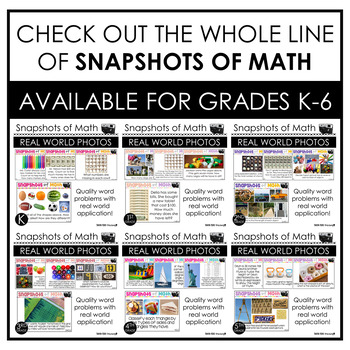# 4th Grade Word Problems Math Spiral Review4th - 5th, Higher Education, Homeschool
Subjects
Standards
Formats Included
• Zip
Pages
140 pages

### Description

Visual representation is important to students. Get your students excited about math by Using Snapshots of Math Word Problems!

This purchase contains 130 word problems for all the 4th grade math standards. Each word problem is applicable for young learners and includes a photo for visual representation. Each question is on a PowerPoint slide for easy use and can be used during a whole group lesson, to introduce a skill, or extra practice.

Standards & Topics Covered

Number and Operation in Base Ten

➥ 4.NB.1 - Place Value Concepts

➥ 4.NBT.2 - Number Form, Word Form, Expanded Form

➥ 4.NBT.3 - Rounding multi-digit whole numbers

➥ 4.NBT.4 - Adding & Subtracting Whole Numbers

➥ 4.NBT.5 - Multiplying whole numbers

➥ 4.NBT.6 - Dividing whole numbers

Operations & Algebraic Thinking

➥ 4.OA.1 - Interpreting multiplication equations

➥ 4.OA.2 - Multiplying and dividing word problems

➥ 4.OA.3 - Solving multistep word problems, including interpreting remainders

➥ 4.OA.4 - Identifying prime and composite numbers within 100

➥ 4.OA.5 - Identifying rules of patterns

Number and Operation - Fractions

➥ 4.NF.1 - Equivalent fractions

➥ 4.NF.2 - Comparing fractions

➥ 4.NF.3 - Adding and subtracting fractions with like denominators, decomposing fractions, adding and subtracting mixed numbers with like denominators

➥ 4.NF. 4 - Multiplying a fraction by a whole number

➥ 4.NF.5 - Adding and subtracting fractions with denominators of 10 and 100

➥ 4.NF.6 - Decimal notation for fractions with denominators of 10 and 100

➥ 4.NF.7 - Comparing decimals to hundredths

Measurement and Data

➥ 4.MD.1 - Measurement and converting measurement

➥ 4.MD.2 - Solving measurement word problems

➥ 4.MD.3 - Area and perimeter of rectangles

➥ 4.MD.4 - Line plots

➥ 4.MD.5 - Angles within a circle

➥ 4.MD.6 - Measuring angles with a protractor

➥ 4.MD.7 - Additive angle measurement, decomposing angles

Geometry

➥ 4.G.1 - Identifying angles in 2D shapes

➥ 4.G.2 - Classifying 2D figures

➥ 4.G.3 - Symmetry

Total Pages
140 pages
Included
Teaching Duration
1 Year
Report this resource to TpT
Reported resources will be reviewed by our team. Report this resource to let us know if this resource violates TpT’s content guidelines.

### Standards

to see state-specific standards (only available in the US).
Interpret a multiplication equation as a comparison, e.g., interpret 35 = 5 × 7 as a statement that 35 is 5 times as many as 7 and 7 times as many as 5. Represent verbal statements of multiplicative comparisons as multiplication equations.
Multiply or divide to solve word problems involving multiplicative comparison, e.g., by using drawings and equations with a symbol for the unknown number to represent the problem, distinguishing multiplicative comparison from additive comparison.
Solve multistep word problems posed with whole numbers and having whole-number answers using the four operations, including problems in which remainders must be interpreted. Represent these problems using equations with a letter standing for the unknown quantity. Assess the reasonableness of answers using mental computation and estimation strategies including rounding.
Find all factor pairs for a whole number in the range 1-100. Recognize that a whole number is a multiple of each of its factors. Determine whether a given whole number in the range 1-100 is a multiple of a given one-digit number. Determine whether a given whole number in the range 1-100 is prime or composite.
Generate a number or shape pattern that follows a given rule. Identify apparent features of the pattern that were not explicit in the rule itself. For example, given the rule “Add 3” and the starting number 1, generate terms in the resulting sequence and observe that the terms appear to alternate between odd and even numbers. Explain informally why the numbers will continue to alternate in this way.

### Questions & Answers

Teachers Pay Teachers is an online marketplace where teachers buy and sell original educational materials.